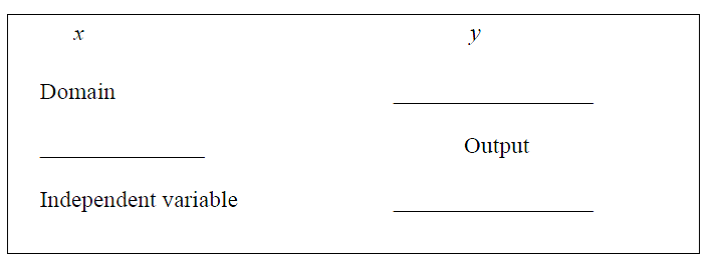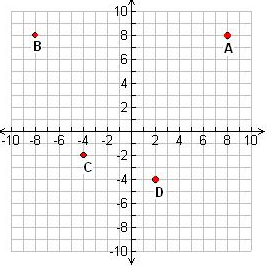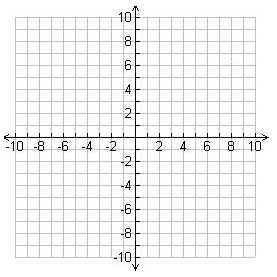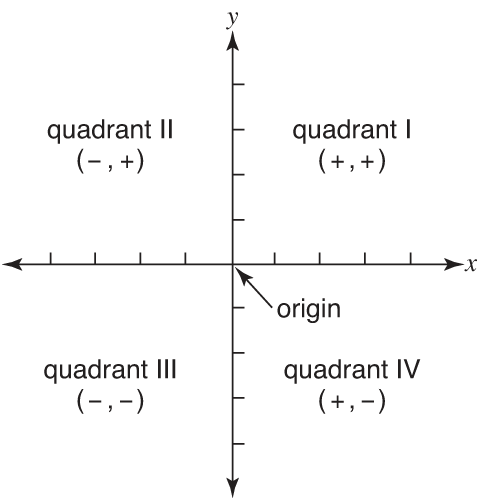# Relationship between two variables#### Everything You Need in One Place

Homework problems? Exam preparation? Trying to grasp a concept or just brushing up the basics? Our extensive help & practice library have got you covered.#### Learn and Practice With Ease

Our proven video lessons ease you through problems quickly, and you get tonnes of friendly practice on questions that trip students up on tests and finals.#### Instant and Unlimited Help

Our personalized learning platform enables you to instantly find the exact walkthrough to your specific type of question. Activate unlimited help now!

0/7
##### Examples
###### Lessons
1. Understanding the difference between X & Y

1.2. Express the relation using mapping notation and ordered pairs.
1. x = { -5 , -3 , -1 , 1 , 3 }
y = { 2 , 4 , 6 , 10 , 12 }
2. x = { -4 , -2 , 0 , 2 , 4 }
y = { 3 , 3 , 3 , 3 , 3 }
3. (1,3) , (2,2) , (3,1)
3. Indicate the quadrant of the following points.
1. (5, -3) (-3, 4) (-4, -3) (2, -6)
4. Plot or find the points on the grid
1. Find the coordinates A, B, C & D points2. Plot the following points on the grid:
(4,5), (-2, 4), (-4,-3) & (5,-6)###### Topic Notes
This lesson will teach us the basic concepts of relationship between two quantities in the coordinate system. For instances, is y a dependent variable or independent variable? What is domain and range? What is an ordered pair? What is quadrant? And how do we plot points on a graph?
Coordinate
System
: A presentation of a real number by points on the real number line using an order pair.

Order
pairs:
A point ( x , y ) on the coordinate plane. Eg. (-3,5), (0,5)…

Origin: The intersection of x-axis and y-axis $\to ( 0 , 0 )$

Relations: Sets of ordered pairs ( x , y )

Domain: All the x-values on the coordinate system

Range: All the y-values on the coordinate system

Independent Variable: The "x" values, which are also known as the inputs when solving for a solution.

Dependent Variable: The "y" values, which are also known as the outputs when solving for a solution.